Скачать презентацию The 3 rd ACM IEEE International Symposium on Networks-on-Chip

a1f0c5df81322d936502c6d21c3a16d1.ppt

• Количество слайдов: 33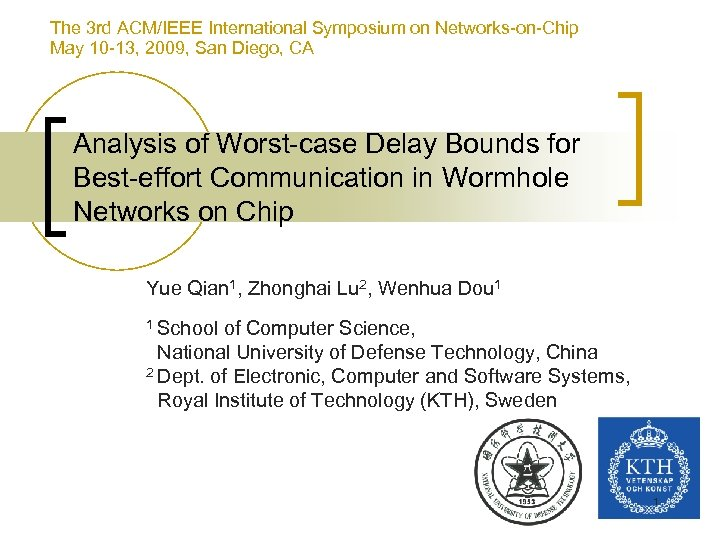The 3 rd ACM/IEEE International Symposium on Networks-on-Chip May 10 -13, 2009, San Diego, CA Analysis of Worst-case Delay Bounds for Best-effort Communication in Wormhole Networks on Chip Yue Qian 1, Zhonghai Lu 2, Wenhua Dou 1 1 School of Computer Science, National University of Defense Technology, China 2 Dept. of Electronic, Computer and Software Systems, Royal Institute of Technology (KTH), Sweden 1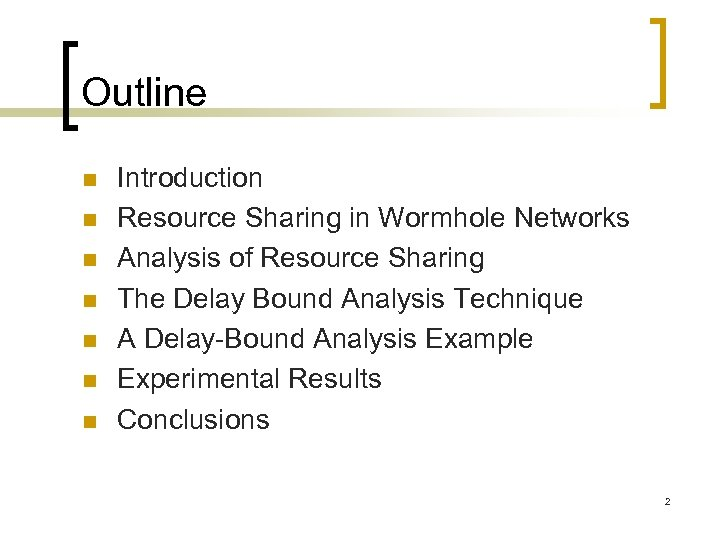Outline n n n n Introduction Resource Sharing in Wormhole Networks Analysis of Resource Sharing The Delay Bound Analysis Technique A Delay-Bound Analysis Example Experimental Results Conclusions 2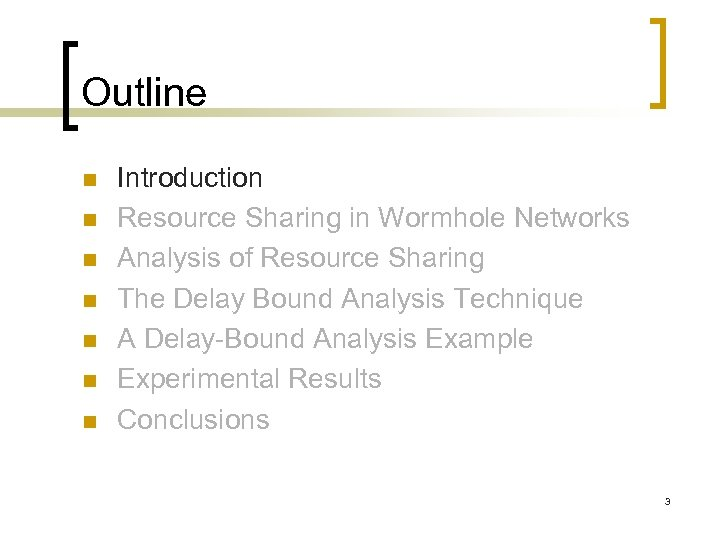Outline n n n n Introduction Resource Sharing in Wormhole Networks Analysis of Resource Sharing The Delay Bound Analysis Technique A Delay-Bound Analysis Example Experimental Results Conclusions 3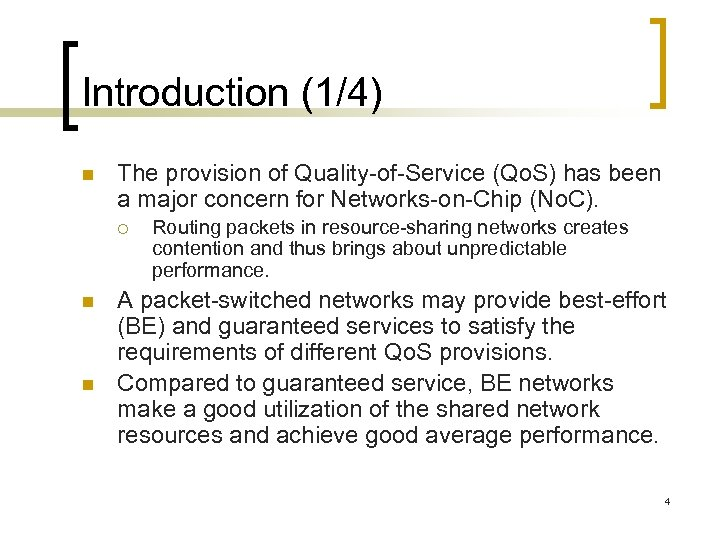Introduction (1/4) n The provision of Quality-of-Service (Qo. S) has been a major concern for Networks-on-Chip (No. C). ¡ n n Routing packets in resource-sharing networks creates contention and thus brings about unpredictable performance. A packet-switched networks may provide best-effort (BE) and guaranteed services to satisfy the requirements of different Qo. S provisions. Compared to guaranteed service, BE networks make a good utilization of the shared network resources and achieve good average performance. 4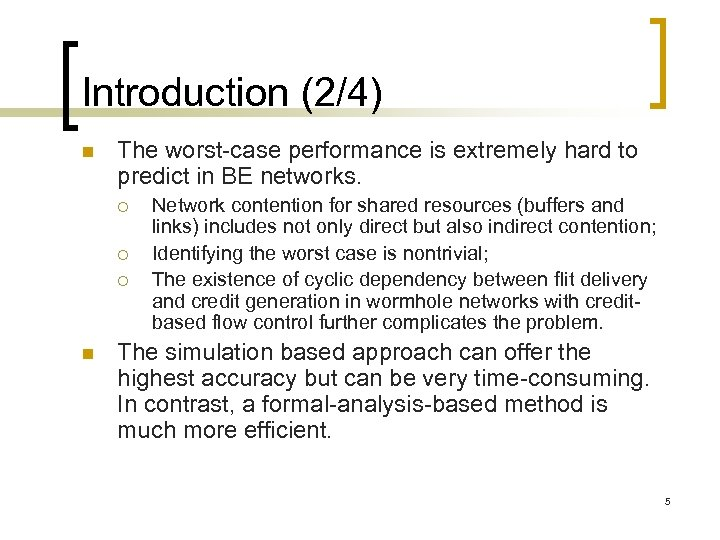Introduction (2/4) n The worst-case performance is extremely hard to predict in BE networks. ¡ ¡ ¡ n Network contention for shared resources (buffers and links) includes not only direct but also indirect contention; Identifying the worst case is nontrivial; The existence of cyclic dependency between flit delivery and credit generation in wormhole networks with creditbased flow control further complicates the problem. The simulation based approach can offer the highest accuracy but can be very time-consuming. In contrast, a formal-analysis-based method is much more efficient. 5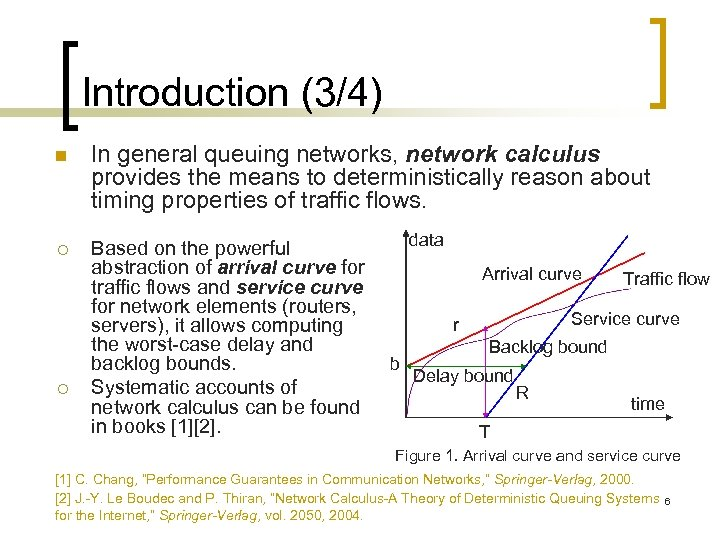Introduction (3/4) n In general queuing networks, network calculus provides the means to deterministically reason about timing properties of traffic flows. ¡ Based on the powerful abstraction of arrival curve for traffic flows and service curve for network elements (routers, servers), it allows computing the worst-case delay and backlog bounds. Systematic accounts of network calculus can be found in books . ¡ data Arrival curve Service curve r b Traffic flow Backlog bound Delay bound R time T Figure 1. Arrival curve and service curve  C. Chang, “Performance Guarantees in Communication Networks, ” Springer-Verlag, 2000.  J. -Y. Le Boudec and P. Thiran, “Network Calculus-A Theory of Deterministic Queuing Systems for the Internet, ” Springer-Verlag, vol. 2050, 2004. 6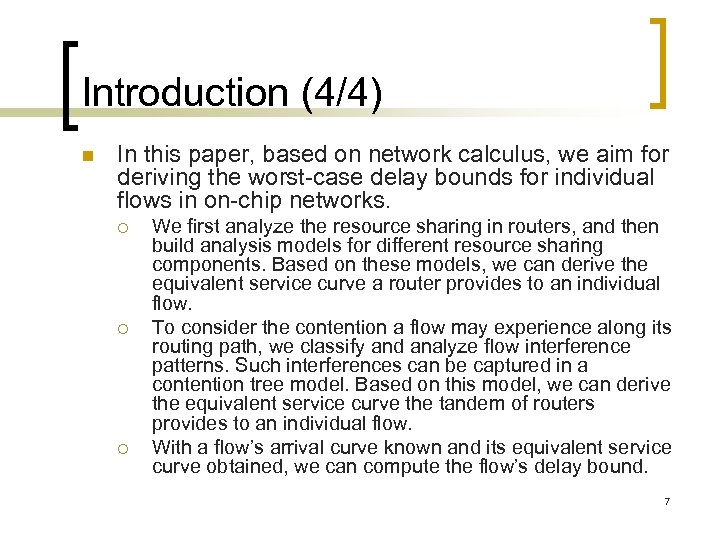Introduction (4/4) n In this paper, based on network calculus, we aim for deriving the worst-case delay bounds for individual flows in on-chip networks. ¡ ¡ ¡ We first analyze the resource sharing in routers, and then build analysis models for different resource sharing components. Based on these models, we can derive the equivalent service curve a router provides to an individual flow. To consider the contention a flow may experience along its routing path, we classify and analyze flow interference patterns. Such interferences can be captured in a contention tree model. Based on this model, we can derive the equivalent service curve the tandem of routers provides to an individual flow. With a flow’s arrival curve known and its equivalent service curve obtained, we can compute the flow’s delay bound. 7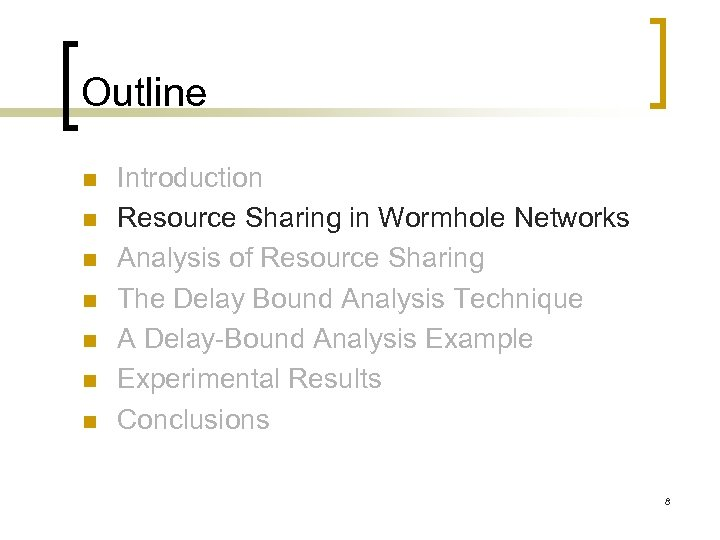Outline n n n n Introduction Resource Sharing in Wormhole Networks Analysis of Resource Sharing The Delay Bound Analysis Technique A Delay-Bound Analysis Example Experimental Results Conclusions 8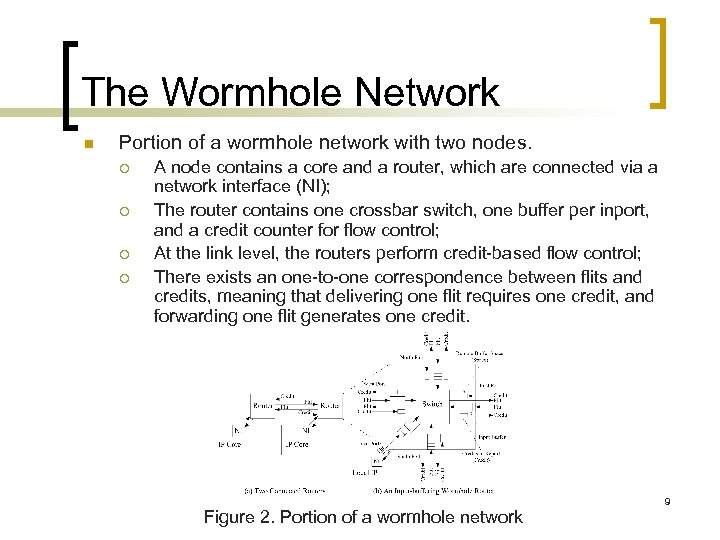The Wormhole Network n Portion of a wormhole network with two nodes. ¡ ¡ A node contains a core and a router, which are connected via a network interface (NI); The router contains one crossbar switch, one buffer per inport, and a credit counter for flow control; At the link level, the routers perform credit-based flow control; There exists an one-to-one correspondence between flits and credits, meaning that delivering one flit requires one credit, and forwarding one flit generates one credit. Figure 2. Portion of a wormhole network 9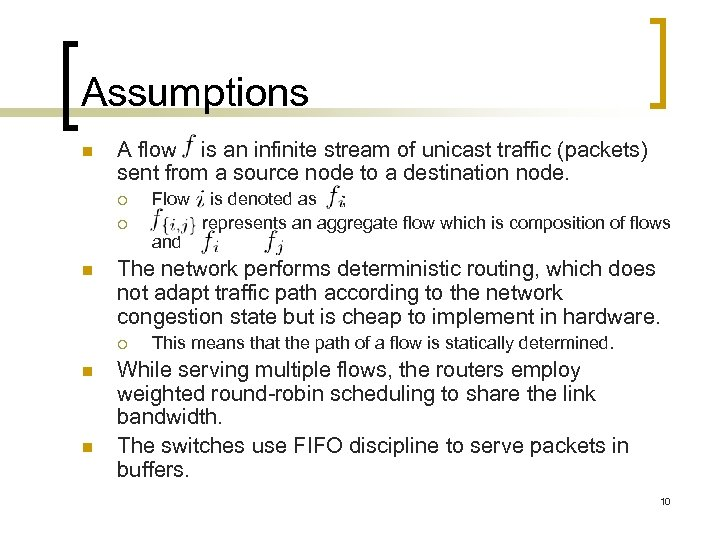Assumptions n A flow is an infinite stream of unicast traffic (packets) sent from a source node to a destination node. ¡ Flow ¡ and n The network performs deterministic routing, which does not adapt traffic path according to the network congestion state but is cheap to implement in hardware. ¡ n n is denoted as ; represents an aggregate flow which is composition of flows. This means that the path of a flow is statically determined. While serving multiple flows, the routers employ weighted round-robin scheduling to share the link bandwidth. The switches use FIFO discipline to serve packets in buffers. 10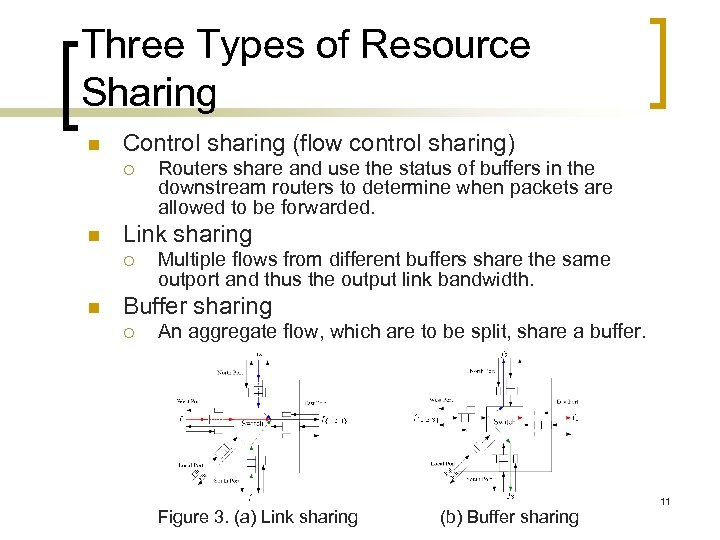Three Types of Resource Sharing n Control sharing (flow control sharing) ¡ n Link sharing ¡ n Routers share and use the status of buffers in the downstream routers to determine when packets are allowed to be forwarded. Multiple flows from different buffers share the same outport and thus the output link bandwidth. Buffer sharing ¡ An aggregate flow, which are to be split, share a buffer. Figure 3. (a) Link sharing (b) Buffer sharing 11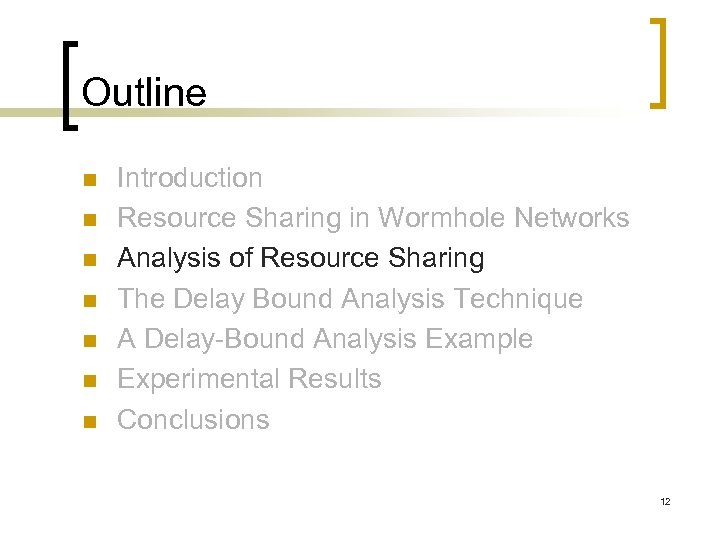Outline n n n n Introduction Resource Sharing in Wormhole Networks Analysis of Resource Sharing The Delay Bound Analysis Technique A Delay-Bound Analysis Example Experimental Results Conclusions 12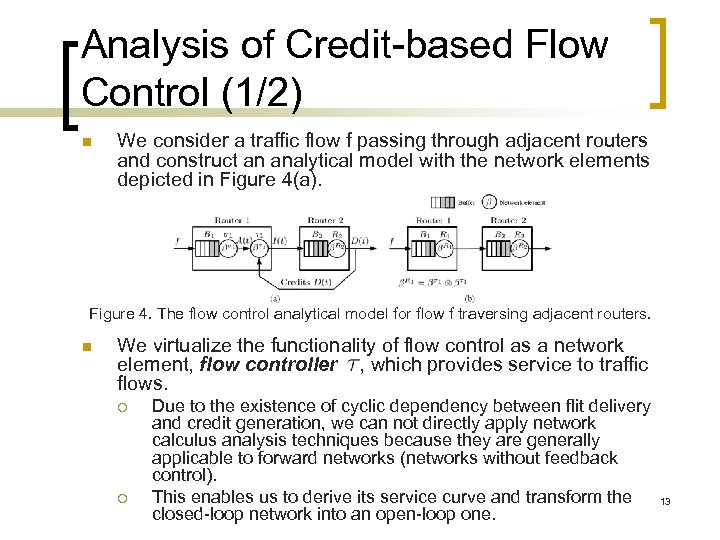Analysis of Credit-based Flow Control (1/2) n We consider a traffic flow f passing through adjacent routers and construct an analytical model with the network elements depicted in Figure 4(a). Figure 4. The flow control analytical model for flow f traversing adjacent routers. n We virtualize the functionality of flow control as a network element, flow controller , which provides service to traffic flows. ¡ ¡ Due to the existence of cyclic dependency between flit delivery and credit generation, we can not directly apply network calculus analysis techniques because they are generally applicable to forward networks (networks without feedback control). This enables us to derive its service curve and transform the closed-loop network into an open-loop one. 13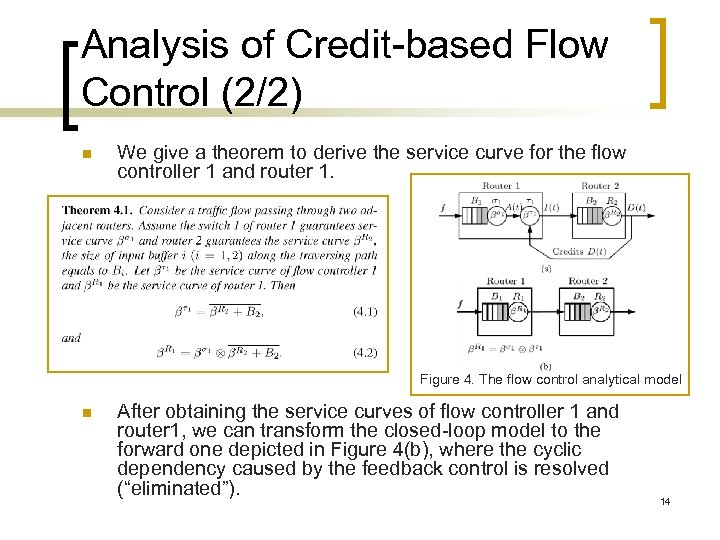Analysis of Credit-based Flow Control (2/2) n We give a theorem to derive the service curve for the flow controller 1 and router 1. Figure 4. The flow control analytical model n After obtaining the service curves of flow controller 1 and router 1, we can transform the closed-loop model to the forward one depicted in Figure 4(b), where the cyclic dependency caused by the feedback control is resolved (“eliminated”). 14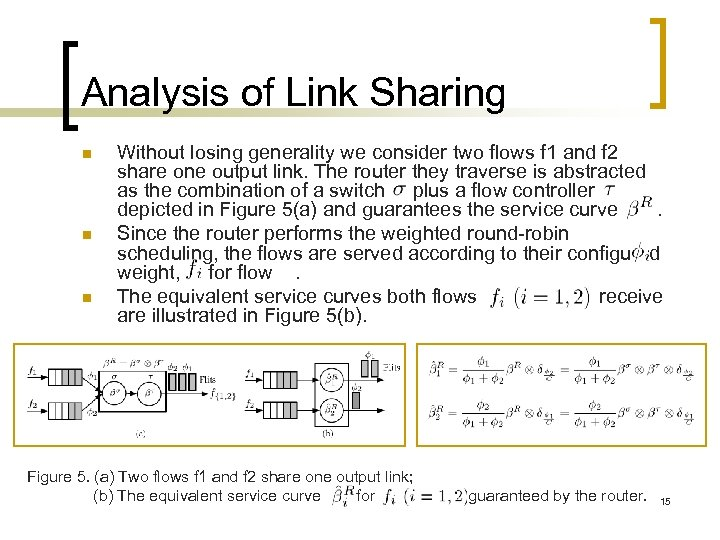Analysis of Link Sharing n n n Without losing generality we consider two flows f 1 and f 2 share one output link. The router they traverse is abstracted as the combination of a switch plus a flow controller depicted in Figure 5(a) and guarantees the service curve. Since the router performs the weighted round-robin scheduling, the flows are served according to their configured weight, for flow. The equivalent service curves both flows receive are illustrated in Figure 5(b). Figure 5. (a) Two flows f 1 and f 2 share one output link; (b) The equivalent service curve for guaranteed by the router. 15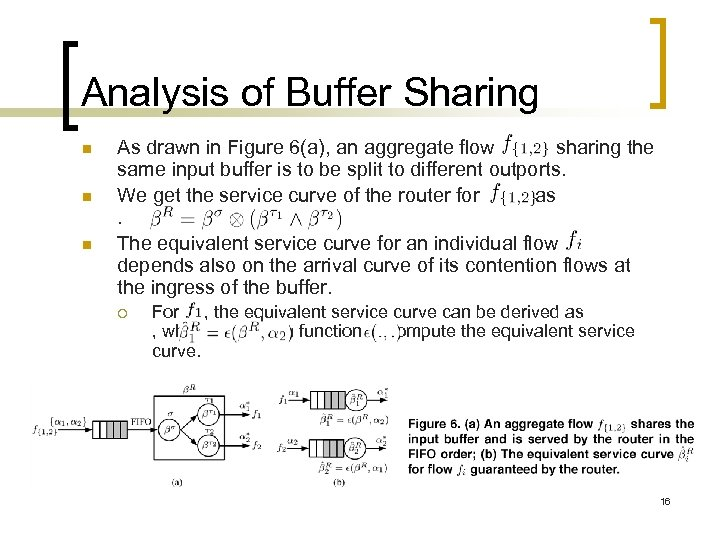Analysis of Buffer Sharing n n n As drawn in Figure 6(a), an aggregate flow sharing the same input buffer is to be split to different outports. We get the service curve of the router for as. The equivalent service curve for an individual flow depends also on the arrival curve of its contention flows at the ingress of the buffer. ¡ For , the equivalent service curve can be derived as , where is a function to compute the equivalent service curve. 16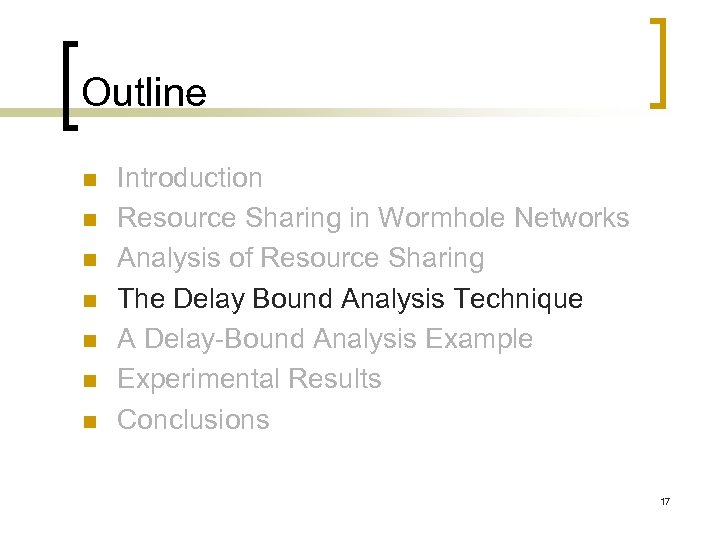Outline n n n n Introduction Resource Sharing in Wormhole Networks Analysis of Resource Sharing The Delay Bound Analysis Technique A Delay-Bound Analysis Example Experimental Results Conclusions 17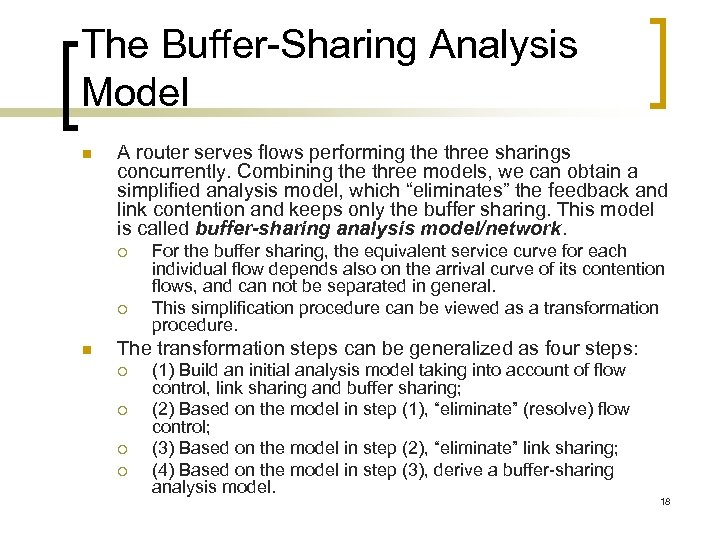The Buffer-Sharing Analysis Model n A router serves flows performing the three sharings concurrently. Combining the three models, we can obtain a simplified analysis model, which “eliminates” the feedback and link contention and keeps only the buffer sharing. This model is called buffer-sharing analysis model/network. ¡ ¡ n For the buffer sharing, the equivalent service curve for each individual flow depends also on the arrival curve of its contention flows, and can not be separated in general. This simplification procedure can be viewed as a transformation procedure. The transformation steps can be generalized as four steps: ¡ ¡ (1) Build an initial analysis model taking into account of flow control, link sharing and buffer sharing; (2) Based on the model in step (1), “eliminate” (resolve) flow control; (3) Based on the model in step (2), “eliminate” link sharing; (4) Based on the model in step (3), derive a buffer-sharing analysis model. 18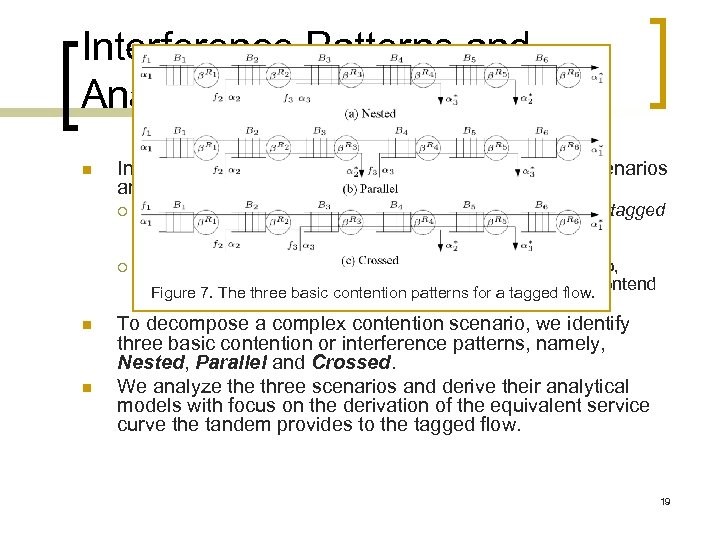Interference Patterns and Analytical Models n In a buffer-sharing analysis network, flow contention scenarios are diverse and complicated. ¡ ¡ n n We call the flow for which we shall derive its delay bound tagged flow, other flows sharing resources with it contention or interfering flows. A tagged flow directly contends with interfering flows. Also, interfering flows may contend with each other and then contend Figure 7. The three basic contention patterns for a tagged flow. with the tagged flow again. To decompose a complex contention scenario, we identify three basic contention or interference patterns, namely, Nested, Parallel and Crossed. We analyze three scenarios and derive their analytical models with focus on the derivation of the equivalent service curve the tandem provides to the tagged flow. 19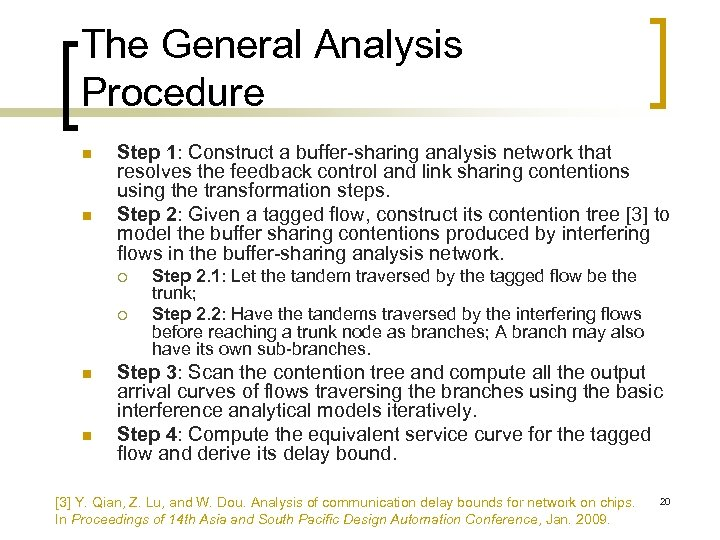The General Analysis Procedure n n Step 1: Construct a buffer-sharing analysis network that resolves the feedback control and link sharing contentions using the transformation steps. Step 2: Given a tagged flow, construct its contention tree  to model the buffer sharing contentions produced by interfering flows in the buffer-sharing analysis network. ¡ ¡ n n Step 2. 1: Let the tandem traversed by the tagged flow be the trunk; Step 2. 2: Have the tandems traversed by the interfering flows before reaching a trunk node as branches; A branch may also have its own sub-branches. Step 3: Scan the contention tree and compute all the output arrival curves of flows traversing the branches using the basic interference analytical models iteratively. Step 4: Compute the equivalent service curve for the tagged flow and derive its delay bound.  Y. Qian, Z. Lu, and W. Dou. Analysis of communication delay bounds for network on chips. In Proceedings of 14 th Asia and South Pacific Design Automation Conference, Jan. 2009. 20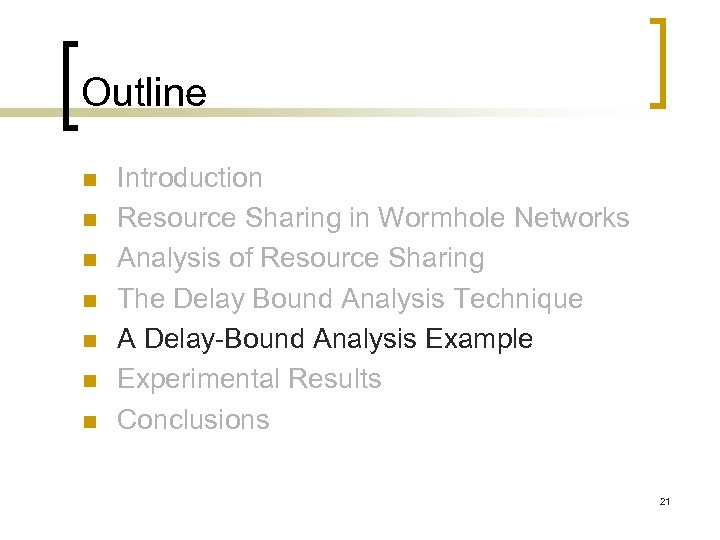Outline n n n n Introduction Resource Sharing in Wormhole Networks Analysis of Resource Sharing The Delay Bound Analysis Technique A Delay-Bound Analysis Example Experimental Results Conclusions 21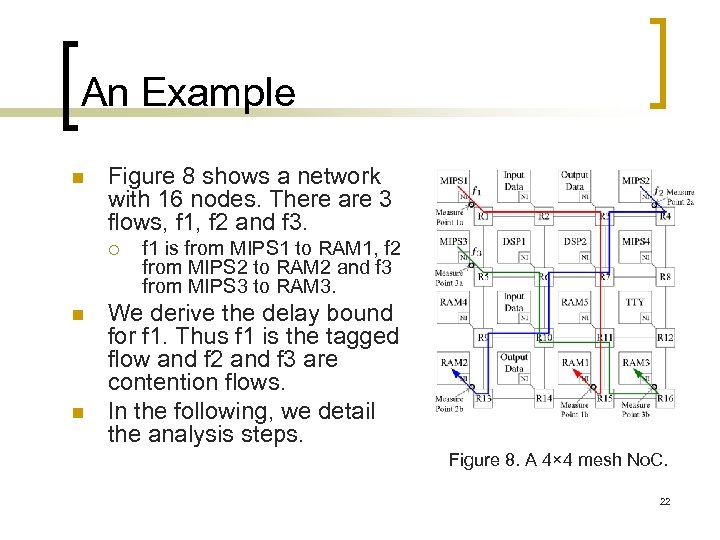An Example n Figure 8 shows a network with 16 nodes. There are 3 flows, f 1, f 2 and f 3. ¡ n n f 1 is from MIPS 1 to RAM 1, f 2 from MIPS 2 to RAM 2 and f 3 from MIPS 3 to RAM 3. We derive the delay bound for f 1. Thus f 1 is the tagged flow and f 2 and f 3 are contention flows. In the following, we detail the analysis steps. Figure 8. A 4× 4 mesh No. C. 22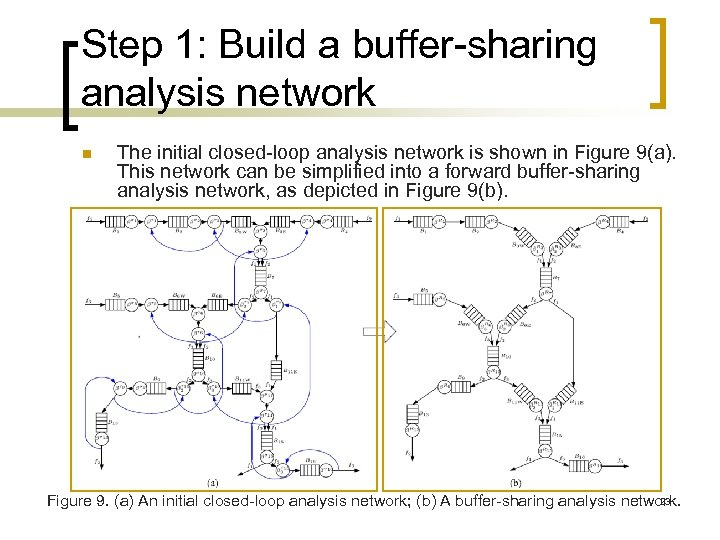Step 1: Build a buffer-sharing analysis network n The initial closed-loop analysis network is shown in Figure 9(a). This network can be simplified into a forward buffer-sharing analysis network, as depicted in Figure 9(b). 23 Figure 9. (a) An initial closed-loop analysis network; (b) A buffer-sharing analysis network.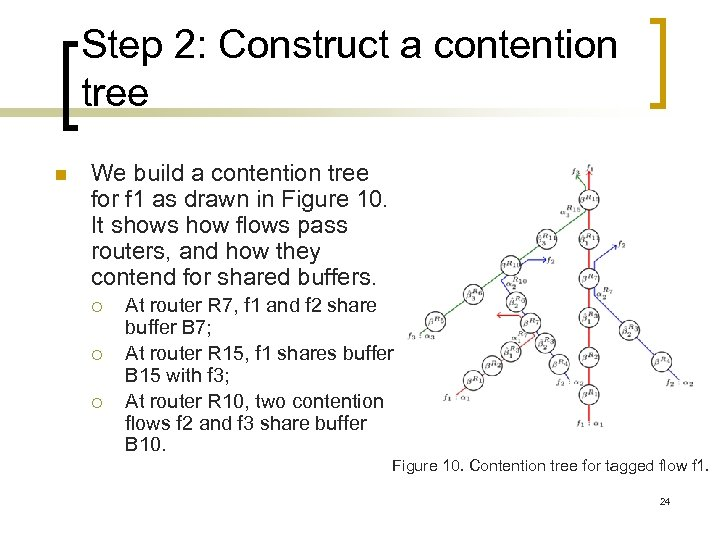Step 2: Construct a contention tree n We build a contention tree for f 1 as drawn in Figure 10. It shows how flows pass routers, and how they contend for shared buffers. ¡ ¡ ¡ At router R 7, f 1 and f 2 share buffer B 7; At router R 15, f 1 shares buffer B 15 with f 3; At router R 10, two contention flows f 2 and f 3 share buffer B 10. Figure 10. Contention tree for tagged flow f 1. 24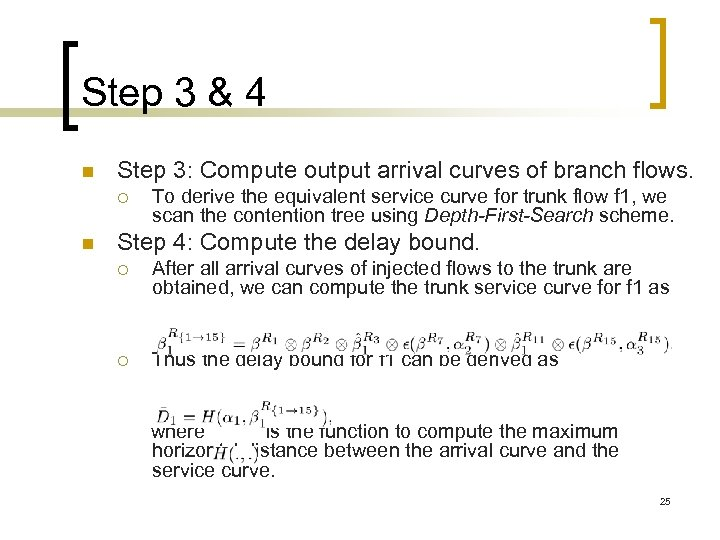Step 3 & 4 n Step 3: Compute output arrival curves of branch flows. ¡ n To derive the equivalent service curve for trunk flow f 1, we scan the contention tree using Depth-First-Search scheme. Step 4: Compute the delay bound. ¡ After all arrival curves of injected flows to the trunk are obtained, we can compute the trunk service curve for f 1 as ¡ Thus the delay bound for f 1 can be derived as where is the function to compute the maximum horizontal distance between the arrival curve and the service curve. 25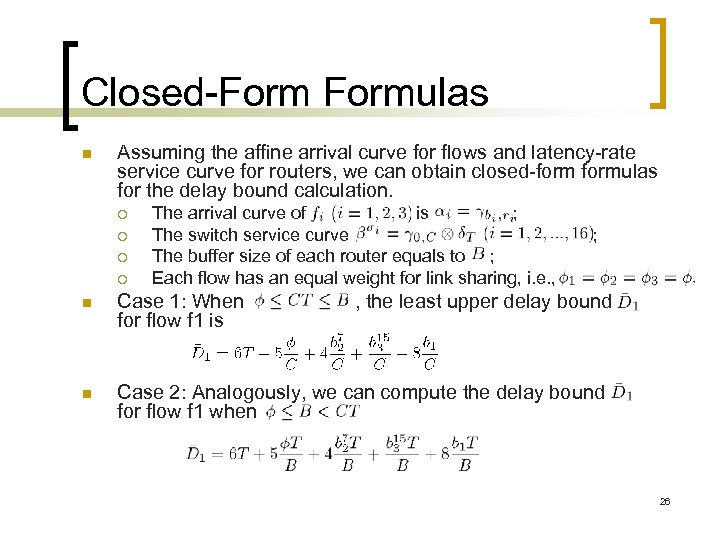Closed-Formulas n Assuming the affine arrival curve for flows and latency-rate service curve for routers, we can obtain closed-formulas for the delay bound calculation. ¡ ¡ The arrival curve of is ; The switch service curve The buffer size of each router equals to ; Each flow has an equal weight for link sharing, i. e. , ; n Case 1: When for flow f 1 is , the least upper delay bound n Case 2: Analogously, we can compute the delay bound for flow f 1 when 26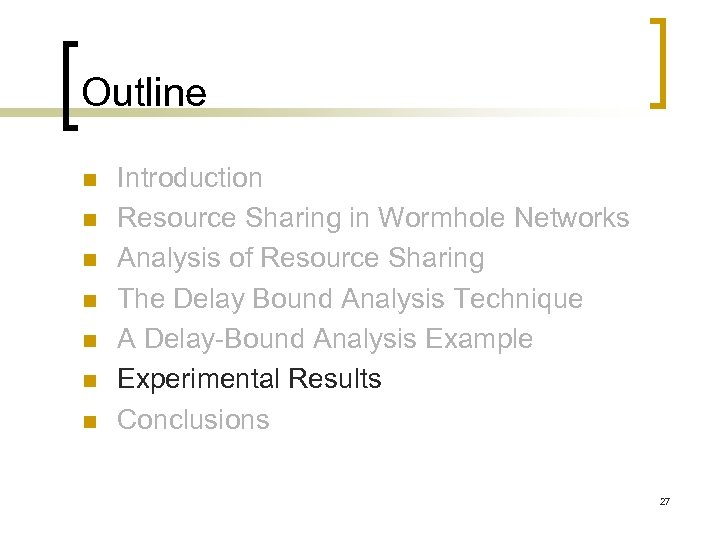Outline n n n n Introduction Resource Sharing in Wormhole Networks Analysis of Resource Sharing The Delay Bound Analysis Technique A Delay-Bound Analysis Example Experimental Results Conclusions 27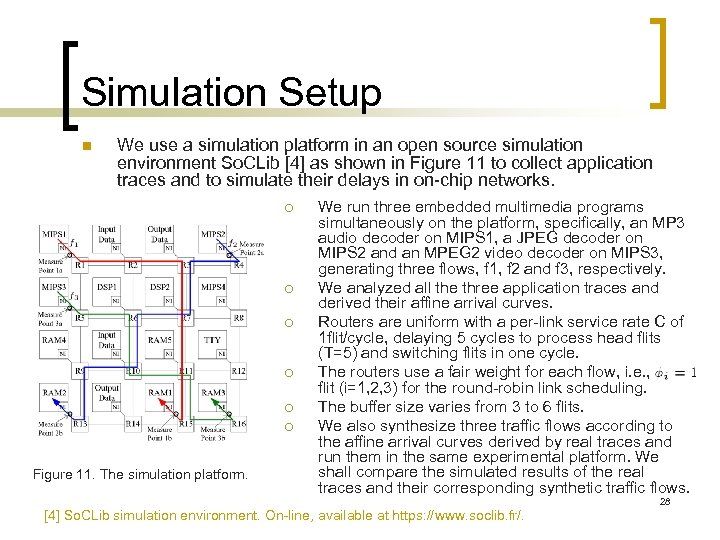Simulation Setup n We use a simulation platform in an open source simulation environment So. CLib  as shown in Figure 11 to collect application traces and to simulate their delays in on-chip networks. ¡ ¡ ¡ Figure 11. The simulation platform. We run three embedded multimedia programs simultaneously on the platform, specifically, an MP 3 audio decoder on MIPS 1, a JPEG decoder on MIPS 2 and an MPEG 2 video decoder on MIPS 3, generating three flows, f 1, f 2 and f 3, respectively. We analyzed all the three application traces and derived their affine arrival curves. Routers are uniform with a per-link service rate C of 1 flit/cycle, delaying 5 cycles to process head flits (T=5) and switching flits in one cycle. The routers use a fair weight for each flow, i. e. , flit (i=1, 2, 3) for the round-robin link scheduling. The buffer size varies from 3 to 6 flits. We also synthesize three traffic flows according to the affine arrival curves derived by real traces and run them in the same experimental platform. We shall compare the simulated results of the real traces and their corresponding synthetic traffic flows.  So. CLib simulation environment. On-line, available at https: //www. soclib. fr/. 28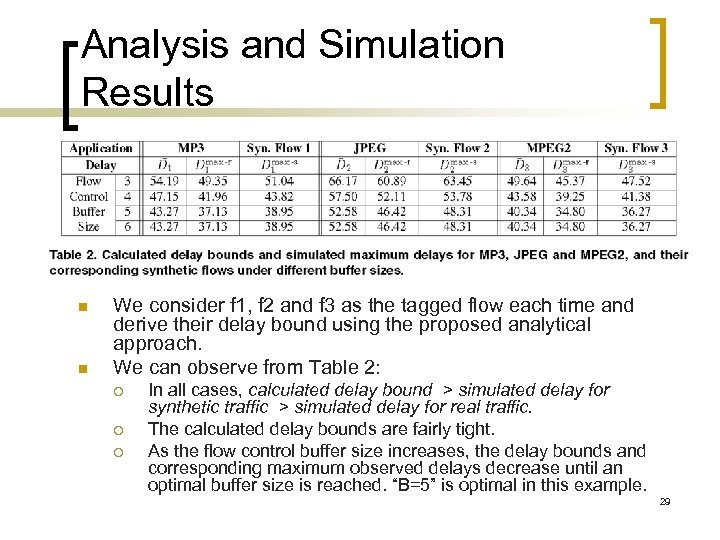Analysis and Simulation Results n n We consider f 1, f 2 and f 3 as the tagged flow each time and derive their delay bound using the proposed analytical approach. We can observe from Table 2: ¡ ¡ ¡ In all cases, calculated delay bound > simulated delay for synthetic traffic > simulated delay for real traffic. The calculated delay bounds are fairly tight. As the flow control buffer size increases, the delay bounds and corresponding maximum observed delays decrease until an optimal buffer size is reached. “B=5” is optimal in this example. 29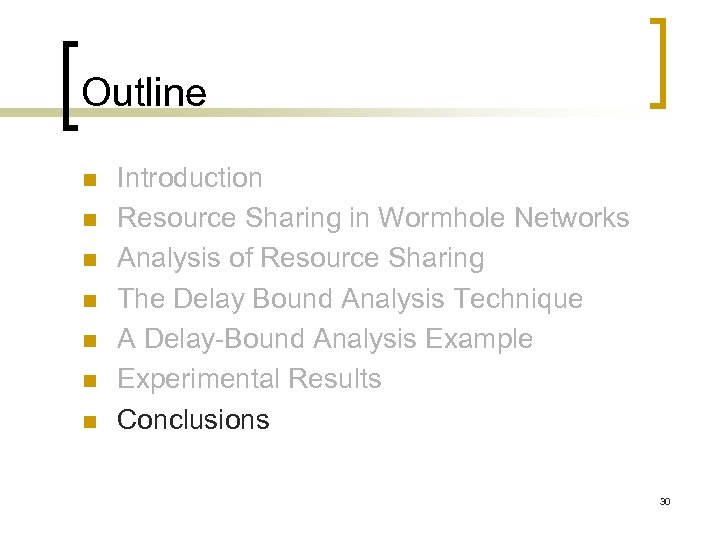Outline n n n n Introduction Resource Sharing in Wormhole Networks Analysis of Resource Sharing The Delay Bound Analysis Technique A Delay-Bound Analysis Example Experimental Results Conclusions 30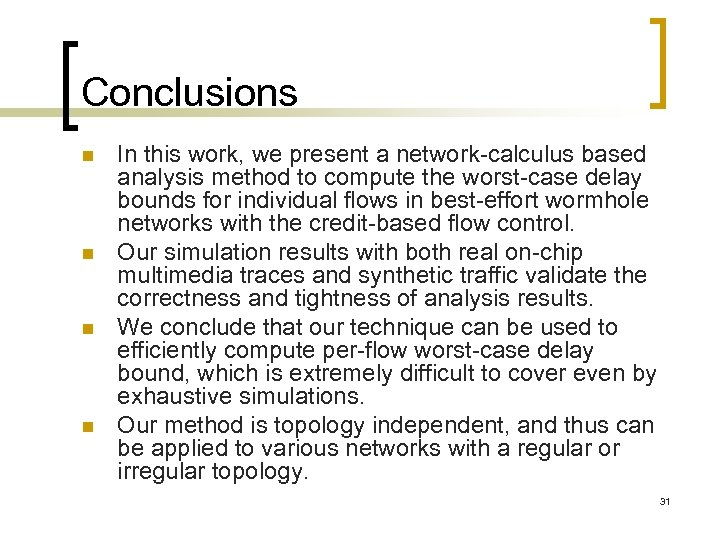Conclusions n n In this work, we present a network-calculus based analysis method to compute the worst-case delay bounds for individual flows in best-effort wormhole networks with the credit-based flow control. Our simulation results with both real on-chip multimedia traces and synthetic traffic validate the correctness and tightness of analysis results. We conclude that our technique can be used to efficiently compute per-flow worst-case delay bound, which is extremely difficult to cover even by exhaustive simulations. Our method is topology independent, and thus can be applied to various networks with a regular or irregular topology. 31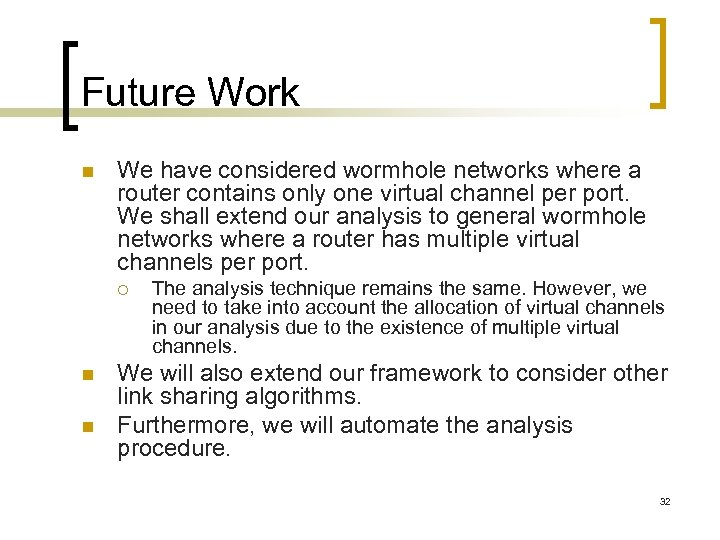Future Work n We have considered wormhole networks where a router contains only one virtual channel per port. We shall extend our analysis to general wormhole networks where a router has multiple virtual channels per port. ¡ n n The analysis technique remains the same. However, we need to take into account the allocation of virtual channels in our analysis due to the existence of multiple virtual channels. We will also extend our framework to consider other link sharing algorithms. Furthermore, we will automate the analysis procedure. 32Any Questions? Thank you very much! 33